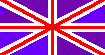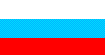CONTENTSVOLUME  2,     issue 2 THEOREM OF CURL OF A POTENTIAL VECTOR IN DYNAMICAL FIELDS Published on 25.04.2002 Here we study the circulation and curl of potential vector in dynamical fields. We prove the theorem that in dynamical fields the curl of potential vector is proportional to the vector product of unit vector of flux direction by the particular derivative of flux of vector with respect to time. With it the vector remains its potential pattern, since the circulation is conditioned by the finite velocity of wave space-propagation. We consider the applications of this theorem to acoustic and electromagnetic fields. We describe the results of experimental studying the transversal acoustic waves in gas medium, which corroborates the possibility to form the transversal wave by way of linear superposition of potential dynamical fields. Keywords: theoretical physics, mathematical physics, wave physics, vector algebra, acoustics, electromagnetic theory, dynamical potential fields. Classification by MSC 2000: 76A02, 76B47, 76N15, 76Q05, 78A02, 78A25, 78A40. Classification by PASC 2001: 03.50.-z; 03.50.De; 41.20.Jb; 43.20.+g; 43.90.+v; 46.25.Cc; 46.40.Cd Full text: / 1 / 2 / 3 / 4 / 5 / 6 / 7 / 8 / 9 / S. B. Karavashkin and O. N. Karavashkina. APPLICATION OF COMPLEX DYNAMICAL MAPPING TO ACOUSTIC FIELDS CHAPTER 1. ACOUSTIC FIELDS PRODUCED BY A SINGLE PULSING SPHERE Published on 27.05.2002 We analyse conventional conformal and alternative non-conformal mapping and prove that this last describes most exactly an acoustic field produced by a single pulsing sphere. By means of dynamical non-conformal mapping we plot the dynamical pattern of process and show that in case of single pulsing sphere the standing wave in the near acoustic field does not arise, as it was thought till now. The inexact estimation of this process is caused by the incorrect comparison of time- and space-phases of process being the argument of trigonometric periodical function. Keywords: Wave physics; Acoustics; Theory of complex variable, Non-conformal mapping, Quasi-conformal mapping Classification by MSC 2000: 30C62; 30C99; 30G30; 32A30; 76-05; 76-99. Classification by PASC 2001: 43.20.+g; 43.38.+n; 43.58.+z; 43.90.+v; 43.20.Hq; 43.20.Tb; 46.25.Cc; 46.40.Cd Full text: / 10 / 11 / 12 / 13 / 14 / 15 / 16 / S. B. Karavashkin and O. N. Karavashkina. THE FEATURES OF LONGITUDINAL COMPRESSION WAVES PROPAGATION IN A FINITE-CROSS-SECTION HOMOGENEOUS ELASTIC ROD (LINEAR MODELLING) Published on 08.08.2002 This paper continues a broad circle devoted to our new method to obtain exact analytical solutions for vibrant elastic systems. Here we present an example of application of this method to a practical problem seeming a simplest at the first sight. However even in this simplest problem the exact analytical solutions reveal quite serious features being unknown or disregarded before. In particular, we prove that, according to the dynamics of process, Poisson coefficient must be negative, in order the rod stretch to correspond its thinning, and vice versa. We also prove that the velocity of accompanying transversal waves propagation is equal to the velocity of longitudinal wave. The same, the velocity of accompanying longitudinal wave is equal to the velocity of transversal wave if inequal velocities of the main longitudinal and transversal waves. We establish that in frames of linear modelling the dynamical variation of a rod density has a non-harmonic periodical pattern; this essentially broadens the conventional range of linear approximation. Classification by MSC 2000: 74B05; 74B10; 74H45; 74J15; 74J30; 74K10; 74S99 Classification by PASC 2001: 46.40.-f; 46.40.Cd; 46.40.Ff; 46.70.Hg Keywords: Elastic distributed lines; Elastic rod vibrations; Surface waves; ODE; Poisson coefficient Full text: / 17 / 18 / 19 / 20 / 21 / 22 / 23 / 24 / 25 / 26 / 27 / S. B. Karavashkin and O. N. Karavashkina. INVESTIGATION OF ELASTIC CONSTRAINT NON-LINEARITY Published in IJMEE, 33 (2005), 2, 116-133 Published on 17.10.2002 This paper is devoted to finding the solution of non-linear vibrations in a homogeneous elastic line consisting of three elements connected by non-linear elastic constraints. The obtained solution is a functional spectral series whose each harmonic is determined analytically on the basis of solution for the system of equations describing the vibration process in one and the same linear system under the forces depending on the degree of elastic constraint non-linearity and on the vibration amplitude of the lowest harmonics. The obtained solutions are analysed. We are revealing that the boundary frequency of each harmonic drops proportionally to the order of harmonic, and resonance spectrum of harmonics of dynamical process contains the spectrum of natural frequencies lower than the natural boundary frequency and the spectrum of frequencies of lower harmonics located between the natural boundary frequency and the boundary frequency of the first harmonic. It is shown that the method of recurrent determination of the spectrum of non-linear dynamical process can be extended to the models with non-linear resistance and to the case of complex-spectrum external force. Classification by MSC 2000: 37N15; 70G60; 70G70; 70K40; 70K75; 74H45; 74J30; 93B18 Classification by PASC 2001: 05.45.-a; 05.45.Tp; 45.20.-d; 45.50.Jf; 45.90.+t Key words: wave physics, mathematical physics, theoretical physics, many-body systems, non-linear dynamics, spectrum of non-linear dynamical process Full text: / 28 / 29 / 30 / 31 / 32 / 33 / 34 / 35 / 36 / 37 / 38 /